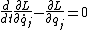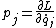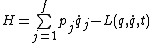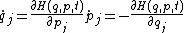# Hamilton's equations of motion

(redirected from Hamiltonian mechanics)
Also found in: Wikipedia.

## Hamilton's equations of motion

The motion of a mechanical system may be described by a set of first-order ordinary differential equations known as Hamilton's equations. Because of their remarkably symmetrical form, they are often referred to as the canonical equations of motion of a system. They are equivalent to Lagrange's equations, but the fact that they are of first order and highly symmetrical makes them advantageous for general discussions of the motion of systems. See Lagrange's equations

Hamilton's equations can be derived from Lagrange's equations. Let the coordinates of the system be qj (j = 1, 2, . . . , f), and let the dynamical description of the system be given by the lagrangian L(q, …, t), where q denotes all the coordinates and a dot denotes total time derivative. Lagrange's equations are then given by Eq. (1). The momentum pj canonically conjugate to qj is defined by Eq. (2).

(1)(2)The hamiltonian H is defined by Eq. (3). Then Hamilton's canonical equations are Eqs. (4).

(3)(4)As they stand, Hamilton's equations are no easier to integrate directly than Lagrange's. Hamilton's equations are of great advantage in more general discussions, and they permit the making of canonical transformations which can lead to simplifications. See Canonical transformations

The hamiltonian function H of classical mechanics is used to form the quantum-mechanical hamiltonian operator.

## Hamilton's equations of motion

[′ham·əl·tənz i¦kwā·zhənz əv ′mō·shən]
(mechanics)
A set of first-order, highly symmetrical equations describing the motion of a classical dynamical system, namely j = ∂ H /∂ pj , j = -∂ H /∂ qj ; here qj (j = 1, 2,…) are generalized coordinates of the system, pj is the momentum conjugate to qj , and H is the Hamiltonian. Also known as canonical equations of motion.
Mentioned in ?
References in periodicals archive ?
They cover fractional Van der Pol oscillation, fractional Lagrangian and Hamiltonian mechanics with memory, fractional relaxation-oscillation phenomena, fractional calculus and long-range interactions, the dynamics of nonlinear systems with power-law memory, fractional viscoelasticity, relationships between one-dimensional and space fractals and fractional integrals and their applications in physics, thermodiffusion in a deformable solid: fractional calculus approach, fractional generalization of gradient mechanics, applying fractional calculus to fractal media, and fractional-order constitutive equations in mechanics and thermodynamics.
He has taught modules in classical mechanics, Lagrangian and Hamiltonian mechanics, nuclear physics, particle physics, quantum field theory, and mathematics for physicists.
Ziglin, "Branching of solutions and nonexistence of first integrals in Hamiltonian mechanics. I," Functional Analysis and Its Applications, vol.
Applications to Hamiltonian Mechanics. Kluwer, Dordrecht, FTPH, 2003.
To study the thermally activated magnetization dynamics of ferromagnetic nanostructures, the authors use the principles of Hamiltonian mechanics to construct a classical microscopic description of an interacting spin system that couples with the surrounding microscopic degrees of freedom.
Baleanu, "Lagrangian and Hamiltonian mechanics on fractals subset of real-line," International Journal of Theoretical Physics, vol.
Hall, Hamiltonian Mechanics and the n-body Problem, Applied Mathematical Sciences, Springer, 1992.
It is shown that classical Lagrangian mechanics is constructed completely on osculating bundles while the levels (higher order tangent bundles) provide a setting for Hamiltonian mechanics.
Sudarshan, Relation between Nambu and Hamiltonian mechanics, Phys.
Aiming to show the connection between classical and quantum mechanics, he first reviews elementary concepts in both areas, including basic math techniques and special functions, Newtonian mechanics, and Schrodinger's wave mechanics; then discusses semiclassical physics, classical periodic orbits, Lagrangian and Hamiltonian mechanics, the phenomenon of chaos, Feynman's Path Integrals, and applications of Gutzwiller's method and the trace formula to quantize chaos.
As far as physics is concerned I shall first deal with the scope of the advances of the theories of classical mechanics, in particular I shall mention only singular advances from Newtonian Mechanics to Lagrangean and to Hamiltonian mechanics. The main purpose of my article is to describe the application of Hamiltonian mechanics to measuring instrument theory.
Applications to Hamiltonian Mechanics, Kluwer, Dordrecht, FTPH 132, 2003.

Site: Follow: Share:
Open / Close